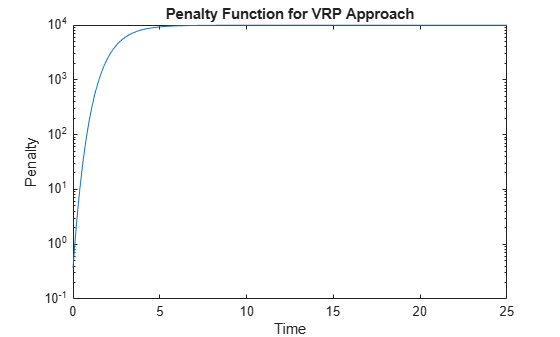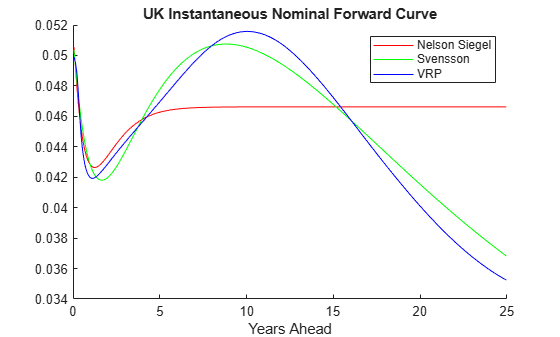# Fitting Interest Rate Curve Functions

This example shows how to use `IRFunctionCurve` objects to model the term structure of interest rates (also referred to as the yield curve). This can be contrasted with modeling the term structure with vectors of dates and data and interpolating between the points (which can currently be done with the function `prbyzero`).

The term structure can refer to at least three different curves: the discount curve, zero curve, or forward curve.

The `IRFunctionCurve` object allows you to model an interest-rate curve as a function.

This example explores using `IRFunctionCurve` to model the default-free term structure of interest rates in the United Kingdom. Three different forms for the term structure are implemented and are discussed in more detail later:

• Nelson-Siegel

• Svensson

• Smoothing Cubic Spline with a so-called Variable Roughness Penalty (VRP)

### Choosing the Data

The first question in modeling the yield curve is what data should be used. To model a default-free yield curve, default-free, option-free market instruments must be used. The most significant component of the data is UK Government Bonds (known as Gilts). Historical data is retrieved from the following site:

https://www.dmo.gov.uk

Repo data is used to construct the short end of the yield curve. Repo data is retrieved from the following site:

https://www.ukfinance.org.uk/

Note also that the data must be specified as a matrix where the columns are `Settle`, `Maturity`, `CleanPrice`, and `CouponRate` and that instruments must be bonds or synthetically converted to bonds.

Market data for a close date of April 30, 2008, has been downloaded and saved to the following data file (`ukdata20080430`), which is loaded into MATLAB® with the following command:

```% Load the data load ukdata20080430 % Convert repo rates to be equivalent zero coupon bonds RepoCouponRate = repmat(0,size(RepoRates)); RepoPrice = bndprice(RepoRates, RepoCouponRate, RepoSettle, RepoMaturity); % Aggregate the data Settle = [RepoSettle;BondSettle]; Maturity = [RepoMaturity;BondMaturity]; CleanPrice = [RepoPrice;BondCleanPrice]; CouponRate = [RepoCouponRate;BondCouponRate]; Instruments = [Settle Maturity CleanPrice CouponRate]; InstrumentPeriod = [repmat(0,6,1);repmat(2,31,1)]; CurveSettle = datenum('30-Apr-2008');```

### Fit Nelson-Siegel Model to Market Data

The Nelson-Siegel model proposes that the instantaneous forward curve can be modeled with the following:

`$f={\beta }_{0}+{\beta }_{1}{e}^{\frac{-m}{\tau }}+{\beta }_{2}{e}^{\frac{-m}{\tau }}\frac{m}{\tau }$`

This can be integrated to derive an equation for the zero curve (see  for more information on the equations and the derivation):

`$s={\beta }_{0}+\left({\beta }_{1}+{\beta }_{2}\right)\frac{\tau }{m}\left(1-{e}^{\frac{-m}{\tau }}\right)-{\beta }_{2}{e}^{\frac{-m}{\tau }}$`

The `IRFunctionCurve` object provides the capability to fit a Nelson Siegel curve to observed market data with the `fitNelsonSiegel` method. The fitting is done by calling the Optimization Toolbox™ function `lsqnonlin`.

The `fitNelsonSiegel` method has required inputs for Curve `Type`, Curve `Settle`, and a matrix of instrument data.

Optional input arguments, specified in name-value pair argument, are:

• `IRFitOptions` structure: Provides the capability to choose which quantity to be minimized (price, yield, or duration weighted price) and other optimization parameters (for example, upper and lower bounds for parameters).

• Curve `Compounding` and `Basis` (day-count convention)

• Additional instrument parameters, `Period`, `Basis`, `FirstCouponDate`, and so on.

```NSModel = IRFunctionCurve.fitNelsonSiegel('Zero',CurveSettle,... Instruments,'InstrumentPeriod',InstrumentPeriod);```

### Fit Svensson Model

A very similar model to the Nelson-Siegel model is the Svensson model, which adds two additional parameters to account for greater flexibility in the term structure. This model proposes that the forward rate can be modeled with the following form:

`$f={\beta }_{0}+{\beta }_{1}{e}^{\frac{-m}{{\tau }_{1}}}+{\beta }_{2}{e}^{\frac{-m}{{\tau }_{1}}}\frac{m}{{\tau }_{1}}+{\beta }_{3}{e}^{\frac{-m}{{\tau }_{2}}}\frac{m}{{\tau }_{2}}$`

As above, this can be integrated to derive an equation for the zero curve:

`$s={\beta }_{0}+{\beta }_{1}\left(1-{e}^{\frac{-m}{{\tau }_{1}}}\right)\left(-\frac{{\tau }_{1}}{m}\right)+{\beta }_{2}\left(\left(1-{e}^{\frac{-m}{{\tau }_{1}}}\right)\frac{{\tau }_{1}}{m}-{e}^{\frac{m}{{\tau }_{1}}}\right)+{\beta }_{3}\left(\left(1-{e}^{\frac{-m}{{\tau }_{2}}}\right)\frac{{\tau }_{2}}{m}-{e}^{\frac{m}{{\tau }_{2}}}\right)$`

Fitting the parameters to this model proceeds in a similar fashion to the Nelson-Siegel model using the `fitSvensson` method.

```SvenssonModel = IRFunctionCurve.fitSvensson('Zero',CurveSettle,... Instruments,'InstrumentPeriod',InstrumentPeriod);```

### Fit Smoothing Spline

The term structure can also be modeled with a spline, specifically, one way to model the term structure is by representing the forward curve with a cubic spline. To ensure that the spline is sufficiently smooth, a penalty is imposed relating to the curvature (second derivative) of the spline:

`$\sum _{i=1}^{N}\left[\frac{{P}_{i}-{\underset{}{\overset{ˆ}{P}}}_{i}\left(f\right)}{{D}_{i}}{\right]}^{2}+{\int }_{0}^{M}{\lambda }_{t}\left(m\right)\left[{f}^{\prime \prime }\left(m\right){\right]}^{2}dm$`

where the first term is the difference between the observed price P and the predicted price, P_hat, (weighted by the bond's duration, D) summed over all bonds in the data set, and the second term is the penalty term (where lambda is a penalty function and f is the spline).

See , ,  below.

There have been different proposals for the specification of the penalty function lambda. One approach, advocated by , and currently used by the UK Debt Management Office, is a penalty function of the following form:

`$log\left(\lambda \left(m\right)\right)=L-\left(L-S\right){e}^{\frac{-m}{\mu }}$`

The parameters L, S, and mu are typically estimated from historical data.

The `IRFunctionCurve` object can be used to fit a smoothing spline representation of the forward curve with a penalty function using the method `fitSmoothingSpline`.

Required inputs, like for the methods above, are a `CurveType`, Curve`Settle`, `Instruments` matrix, and a function handle (`Lambdafun`) containing the penalty function.

The optional parameters are similar to `fitNelsonSiegel` and `fitSvensson`.

```% Parameters chosen to be roughly similar to  below. L = 9.2; S = -1; mu = 1; lambdafun = @(t) exp(L - (L-S)*exp(-t/mu)); % Construct penalty function t = 0:.1:25; % Construct data to plot penalty function y = lambdafun(t); figure semilogy(t,y); title('Penalty Function for VRP Approach') ylabel('Penalty') xlabel('Time')``````VRPModel = IRFunctionCurve.fitSmoothingSpline('Forward',CurveSettle,... Instruments,lambdafun,'Compounding',-1,... 'InstrumentPeriod',InstrumentPeriod);```

### Use Fitted Curves and Plot Results

Once a curve is constructed, methods are used to extract the Forward and Zero Rates and the Discount Factors. This curve can also be converted into a `RateSpec` structure using the `toRateSpec` method. The `RateSpec` can then be used with many other functions in the Financial Instruments Toolbox™

```PlottingDates = CurveSettle+20:30:CurveSettle+365*25; TimeToMaturity = yearfrac(CurveSettle,PlottingDates); NSForwardRates = NSModel.getForwardRates(PlottingDates); SvenssonForwardRates = SvenssonModel.getForwardRates(PlottingDates); VRPForwardRates = VRPModel.getForwardRates(PlottingDates); figure hold on plot(TimeToMaturity,NSForwardRates,'r') plot(TimeToMaturity,SvenssonForwardRates,'g') plot(TimeToMaturity,VRPForwardRates,'b') title('UK Instantaneous Nominal Forward Curve') xlabel('Years Ahead') legend({'Nelson Siegel','Svensson','VRP'})```https://www.bankofengland.co.uk

### Bibliography

This example is based on the following papers and journal articles:

 Nelson, C.R., Siegel, A.F., (1987), "Parsimonious Modelling of Yield Curves." Journal of Business. 60, pp 473-89.

 Svensson, L.E.O. (1994), "Estimating and Interpreting Forward Interest Rates: Sweden 1992-4." International Monetary Fund, IMF Working Paper, 1994/114.

 Fisher, M., Nychka, D., Zervos, D. (1995), "Fitting the Term Structure of Interest Rates with Smoothing Splines." Board of Governors of the Federal Reserve System, Federal Reserve Board Working Paper, 95-1.

 Anderson, N., Sleath, J. (1999), "New Estimates of the UK Real and Nominal Yield Curves." Bank of England Quarterly Bulletin. November, pp 384-92.

 Waggoner, D. (1997), "Spline Methods for Extracting Interest Rate Curves from Coupon Bond Prices." Federal Reserve Board Working Paper, 97-10.

 "Zero-Coupon Yield Curves: Technical Documentation." BIS Papers No. 25, October 2005.

 Bolder, D.J., Gusba,S (2002), "Exponentials, Polynomials, and Fourier Series: More Yield Curve Modelling at the Bank of Canada." Working Papers 02-29, Bank of Canada.

 Bolder, D.J., Streliski, D (1999), "Yield Curve Modelling at the Bank of Canada." Technical Reports 84, Bank of Canada.

﻿